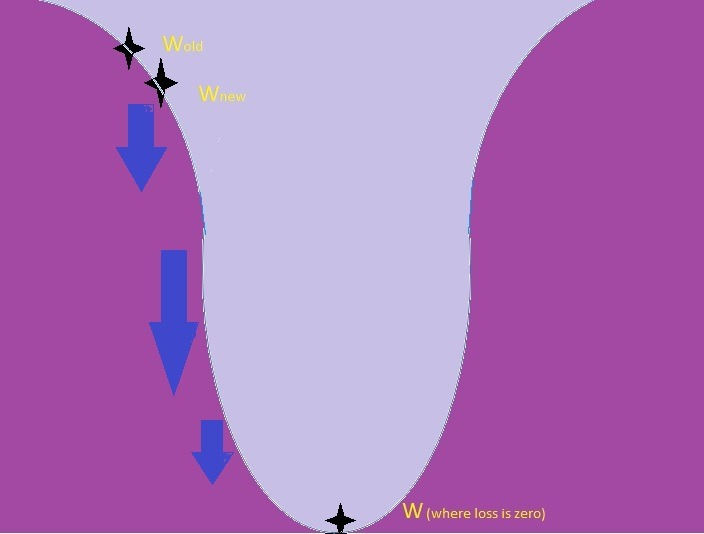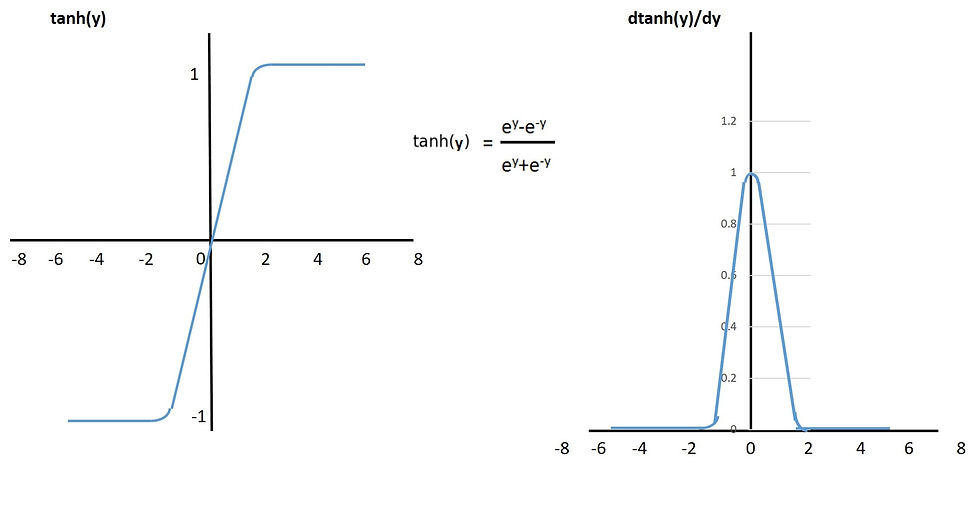top of pageSearch

# Neural Network and its functionality

A neural network is an attempt to replicate human brain and its network of neurons. An ANN artificial neural network is made up of artificial neurons or nodes. An ANN is basically applied for solving artificial intelligence (AI) problems.

As a human brain learns from the information given to it, neural network also does the same.

The connections between neurons are modeled as weights. A positive is referred as an exciting connection, while negative values mean avoiding connections.In neural network work is done in 2 steps:

1) All inputs are multiplied by a weight and summed. This activity is similar to linear equation and a bias is added to it as b. y=∑xw+b.

2) An activation function is applied to the output which decides whether this neuron will be active or not in final decision making. For example, an acceptable range of output is usually between 0 and 1, or it could be −1 and 1 or a 0 or 1 depending on the activation function used which may be Sigmoid, tanh or ReLu.These artificial networks can be used for predictive modeling, where it can be trained on a dataset. Self-learning resulting from experience can occur within networks, which can help in deriving conclusions on important problems which was not visibly in dataset.

Applications of Neural Network

Neural Networks are used in various fields of AI like:

1) Predictive modelling of financial data with time series.

2) Classification in pattern and sequence recognition.

3) Clustering and filtering.

A simple neural network has a input layer, hidden layer and output layer. A more complex neural network or a deep multi-layered network can have a large number of hidden layers in which calculation of summation of product of inputs with weights and bias are done, and on second step activation is carried out with respect to function used.

Here I am going to explain about a 3 hidden layered network, with forward propagation and backward propagation.

Forward Propagation in neural networkIn forward propagation input coming from each input cell is loaded with some weight. In each hidden neuron layer 2 steps are done:

1) x1 *w1 +b1, product of weight and input is taken and added to bias b.

2) Activation function is applied to the output in step 1. o1=Act(x1 *w1 +b1).

In hidden layer 2 input will be output from layer 1, i.e. o1 and it will be multiplied by w2 for upper neuron and w3 for lower neuron and bias is added to each.

o2=Act(o1*w2+b2) and o3=Act(o1*w3+b3)

In hidden layer 3, 2 inputs will be coming as o2 and o3 , these will be multiplied with weights w5 and w4 respectively and added.

o5= Act((o2* w5 + o3*w4) +b4)

Y=Act(o5*w6+b5)

Loss is calculated as y-y^, considering simple subtraction of predicted and actual value.

Backward Propagation in neural networkWe do backward propagation in a neural network to find the updated weight value which in turn helps in minimizing loss.

It is also a way of propagating the back into the neural network to know how much of the loss every node is responsible for, and in turn updating of weights in such a way that minimizes the loss by giving the nodes higher or lower weights.

The gradient is a numeric calculation which helps us to know how to adjust the parameters of a network in such a way that its output deviation is minimized and it reached global minima.The algorithm used to effectively train a neural network is through chain rule. It, after each forward pass through a neural network, backpropagation performs a backward pass while adjusting the model's weights and biases.Activation Functions

In artificial neural networks the output of a node depends upon activation function, which in turn makes a node On or Off, less active or more active depending on the type of function used.

Here we will talk about few of most commonly used activation functions like, Sigmoid, tanh and ReLu.

Sigmoid Activation function

It is a mathematical function having a characteristic "S"-shaped curve or sigmoid curve. A common example of a sigmoid function is the logistic function.

Formula is given in the figure.

This function makes sure to keep the value of outcome of a neuron in between 0 to 1.

Its derivation however ranges in between 0 to 0.25.This function can be used as activation function in neural networks which are not very deep, i.e. in which the hidden layers are not large in number as, if the layers are more it will result in vanishing gradient problem.

Vanishing gradient problem is when the value of (ƞ "ƌL" /("ƌ" W1old)) in equation W1 new =W1 old ƞ "ƌL" /("ƌ" W1old) is so small, that the resulting new weight is almost similar to the old weight and hence no weight updating. This results in value in gradient descent being at the same place which doesn’t progresses towards global minima.

Following equation shows how vanishing gradient is a problem.Tanh Activation Function

This activation function has similar functionality as per sigmoid, its formula is as under. In this function value of output comes in between -1 to 1 and its derivation ranges from 0 to 1.

It also has vanishing gradient problem as explained above but it is better than Sigmoid.

However on working with very deep neural networks it also starts giving problem.ReLu Activation Function

In this function the value of output is either 0 or 1, which means it either deactivates neuron or activates it.

Its formula is simple and is given as under.

This doesn’t have vanishing gradient problem as the number is either 0 or 1 but it may result in dead neurons.Conclusion

I hope after reading this article a lot of things must be clear regarding neural network , its working and why are these required.

Also the most commonly used activation functions and their shortcoming as well as strengths.

This was just an overview of the things to keep it simple for learners.